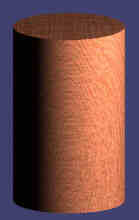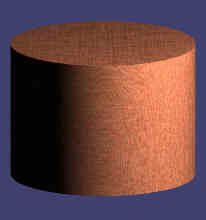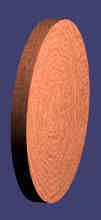# 圆柱体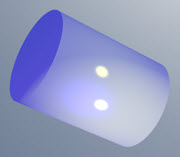• 有平的底面和
平的顶面
• 底面和顶面是一样的
• 从底面到顶面的形状是不变的
• 有一个曲面
• 不是多面体，因为有个曲面## 圆柱体的表面积

• 一端的表面积 = π × r2
• 侧面的表面积 = 2 × π × r × h

### 例子：h = 7，r = 2

 表面积 = 2 × π × r × (r+h) = 2 × π × 2 × (2+7) = 2 × π × 2 × 9 = 36 π ≈ 113.097

## 圆柱体的体积

• 底面积：π × r2
• 高：h
• 体积 = 面积 × 高 = π × r2 × h

### 例子：h = 7，r = 2

 体积 = π × r2 × h = π × 22 × 7 = 28 π ≈  87.96

### 怎样去记：体积 = pizza （披萨的英语）（通常圆周率 "pi" 是写成 πz × z 写成 z2，但整个英语字拼出来比较好记！）

## 圆锥与圆柱的体积圆柱的体积是： π × r2 × h 圆锥的体积是： 1 3 π × r2 × h

## 不一定是圆形的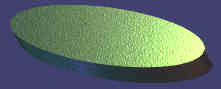## 其它多圆柱体